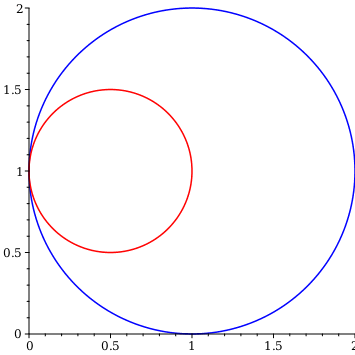Over a Box - Maple HelpSurface Integration over the Surface of a BoxDescription Formulate and evaluate a surface integral for a function defined on the six faces of a box.



Surface Integral on a Box in ${\mathrm{ℝ}}^{3}$

 Integrand $f\left(x,y,z\right)=$${x}_{1}=$$\le x\le$$={x}_{2}$${y}_{1}=$$\le y\le$$={y}_{2}$${z}_{1}=$$\le z\le$$={z}_{2}$Commands UsedSee Also
 >
 >